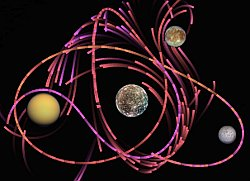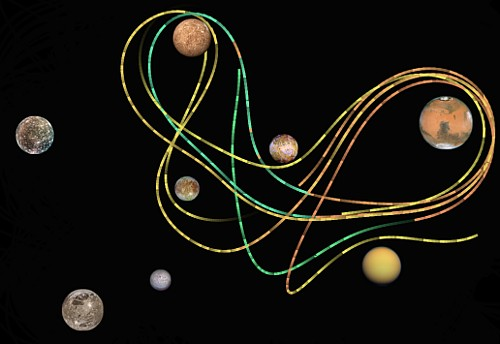# converGIt was about time I learned my way around the HTML5 canvas, so against all reason I wrote an EA in Javascript.

Check it out.

The simulation you see is very simple: independent, inertial particles free-fall in 2-dimensional space at the mercy of the gravity of a handful of fixed bodies.

The individual particles I’ll call “Orbiters”. Each Orbiter is defined by an initial position and velocity. Initial velocities have a fixed magnitude, so they’re represented by a single value: absolute angle. The algorithm optimizes these initial values such that the resulting free-fall trajectory runs very long before hitting a planetary body or going out of bounds.

The Orbiters themselves are not the locus of the evolution. The EA’s population is actually made up of “Waves” which specify the Orbiters’ properties. A Wave is defined by a fixed initial position, base initial angle, and variance in initial angle. The angle and its variance are the only two values which are mutated and evolved, and each Wave is evaluated via a statistically significant number of Orbiters with velocities taken from the normal distribution specified.

Waves reproduce asexually. When a new Wave is created from a parent:

• Initial position is copied exactly
• Base angle is taken from the parent’s longest-running Orbiter and mutated
• In most cases angle variance is greatly reduced, but there is a small chance of the variance being multiplied several times

It progresses like so:

At first there’s variety in initial position and large variance in initial velocity:The population converges to a select few initial positions as variances narrow:Eventually a single initial position dominates, and initial velocity is finely tuned:If the position and velocity converged-on are a lucky choice, this system can optimize the velocity down to the limits of the simulation’s precision. That makes this thing more than a fun gizmo; it’s a successful proof-of-concept for the core technique of an AI for an artillery game with very complex gravity.